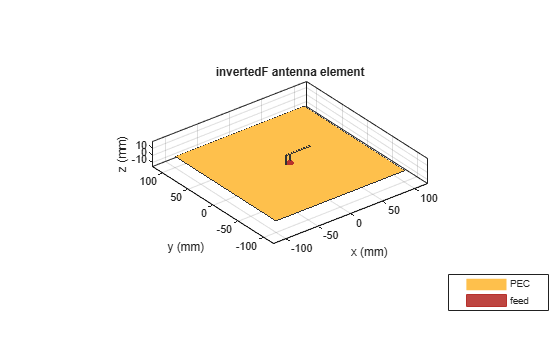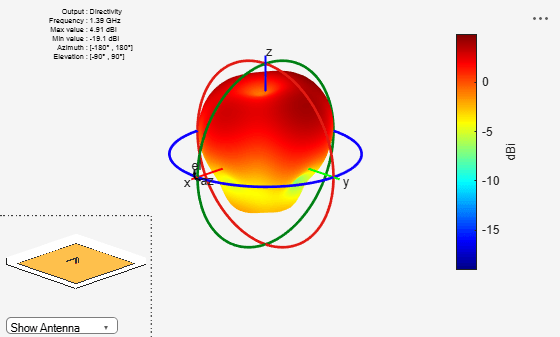# invertedF

Create inverted-F antenna over rectangular ground plane

## Description

The `invertedF` object is an inverted-F antenna mounted over a rectangular ground plane.

The width of the metal strip is related to the diameter of an equivalent cylinder by the equation

`$w=2d=4r$`

where:

• d is the diameter of equivalent cylinder

• r is the radius of equivalent cylinder

For a given cylinder radius, use the utility function `cylinder2strip` to calculate the equivalent width. The default inverted-F antenna is center-fed. The feed point coincides with the origin. The origin is located on the xy- plane.## Creation

### Syntax

``f = invertedF``
``f = invertedF(Name=Value)``

### Description

``` `f = invertedF` creates an inverted-F antenna mounted over a rectangular ground plane. By default, the dimensions are chosen for an operating frequency of 1.7 GHz.```

example

``` `f = invertedF(Name=Value)` creates an inverted-F antenna, with additional Properties specified by one or more name-value arguments. `Name` is the property name and `Value` is the corresponding value. You can specify several name-value pair arguments in any order as `Name1=Value1`,..., `NameN=ValueN`. Properties that you do not specify retain their default values.```

## Properties

expand all

Vertical element height along z-axis, specified a scalar in meters.

Example: `3`

Data Types: `double`

Strip width, specified as a scalar in meters. Strip width must be less than `'Height'`/4 and greater than `'Height'`/1001. 

Example: `0.05`

Data Types: `double`

Stub length from feed to open end, specified as a scalar in meters.

Example: `0.05`

Stub length from feed to shorting end, specified as a scalar in meters.

Example: `0.0050`

Ground plane length along x-axis, specified as a scalar in meters.

Note

Infinite ground plane length is currently unsupported.

Example: `4`

Data Types: `double`

Ground plane width along y-axis, specified as a scalar in meters.

Note

Infinite ground plane width is currently unsupported.

Example: `2.5`

Data Types: `double`

Signed distance from center along length and width of ground plane, specified as a two-element vector.

Example: `[2 1]`

Data Types: `double`

Type of the metal used as a conductor, specified as a metal material object. You can choose any metal from the `MetalCatalog` or specify a metal of your choice. For more information, see `metal`. For more information on metal conductor meshing, see Meshing.

Example: `metal("Copper")`

Lumped elements added to the antenna feed, specified as a `lumpedElement` object.

Example: `lumpedElement(Impedance=75)`

Tilt angle of the antenna in degrees, specified as a scalar or vector. For more information, see Rotate Antennas and Arrays.

Example: `90`

Example: `Tilt=[90 90]`,`TiltAxis=[0 1 0;0 1 1]` tilts the antenna at 90 degrees about the two axes defined by the vectors.

Data Types: `double`

Tilt axis of the antenna, specified as one of these values:

• Three-element vector of Cartesian coordinates in meters. In this case, each coordinate in the vector starts at the origin and lies along the specified points on the x-, y-, and z-axes.

• Two points in space, specified as a 2-by-3 matrix corresponding to two three-element vectors of Cartesian coordinates. In this case, the antenna rotates around the line joining the two points.

• `"x"`, `"y"`, or `"z"` to describe a rotation about the x-, y-, or z-axis, respectively.

Example: `[0 1 0]`

Example: `[0 0 0;0 1 0]`

Example: `"Z"`

Data Types: `double` | `string`

## Object Functions

 `show` Display antenna, array structures, shapes, or platform `info` Display information about antenna or array `axialRatio` Axial ratio of antenna `beamwidth` Beamwidth of antenna `charge` Charge distribution on antenna or array surface `current` Current distribution on antenna or array surface `design` Design prototype antenna or arrays for resonance around specified frequency or create AI-based antenna from antenna catalog objects `efficiency` Radiation efficiency of antenna `EHfields` Electric and magnetic fields of antennas or embedded electric and magnetic fields of antenna element in arrays `impedance` Input impedance of antenna or scan impedance of array `mesh` Mesh properties of metal, dielectric antenna, or array structure `meshconfig` Change meshing mode of antenna, array, custom antenna, custom array, or custom geometry `optimize` Optimize antenna or array using SADEA optimizer `pattern` Plot radiation pattern and phase of antenna or array or embedded pattern of antenna element in array `patternAzimuth` Azimuth plane radiation pattern of antenna or array `patternElevation` Elevation plane radiation pattern of antenna or array `rcs` Calculate and plot radar cross section (RCS) of platform, antenna, or array `returnLoss` Return loss of antenna or scan return loss of array `sparameters` Calculate S-parameters for antennas and antenna arrays `vswr` Voltage standing wave ratio (VSWR) of antenna or array element

## Examples

collapse all

Create and view an inverted-F antenna with 14 mm height over a ground plane of dimensions 200 mm-by-200 mm.

```f = invertedF(Height=14e-3, GroundPlaneLength=200e-3,... GroundPlaneWidth=200e-3); show(f)```This example shows you how to plot the radiation pattern of an inverted-F antenna for a frequency of 1.3 GHz.

```f = invertedF(Height=14e-3, GroundPlaneLength=200e-3,... GroundPlaneWidth=200e-3); pattern(f,1.39e9)```Balanis, C.A. Antenna Theory. Analysis and Design, 3rd Ed. New York: Wiley, 2005.

 Volakis, John. Antenna Engineering Handbook, 4th Ed. New York: Mcgraw-Hill, 2007.